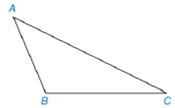Chapter 7.1, Problem 5EElementary Geometry For College St...

7th Edition
Alexander + 2 others
ISBN: 9781337614085

Solutions

Chapter
SectionElementary Geometry For College St...

7th Edition
Alexander + 2 others
ISBN: 9781337614085
Textbook Problem

In Exercises 3 to 8, use the drawing provided.Given: Obtuse ∆ A B C Construct: The perpendicular bisector of A B -

To determine

To construct:

The perpendicular bisector of AB- for the obtuse ABC as shown in the given figure.

Explanation

Definition:

Perpendicular bisector of a triangle is a line passing through the midpoint of the particular side of the triangle.

Calculation:

The given obtuse ABC is shown below:

To construct a bisector we follow the below procedure.

Step 1: Using the compass, take a length of two-third of the length of AB and take A as centre, draw an arc inside of the triangle.

Step 2: Similarly, take B as centre, draw an arc in the same side of arc drawn earlier to obtain a point D

Still sussing out bartleby?

Check out a sample textbook solution.

See a sample solution

The Solution to Your Study Problems

Bartleby provides explanations to thousands of textbook problems written by our experts, many with advanced degrees!

Get Started

In Exercises 107-120, factor each expression completely. 115. 12x2 2x 30

Applied Calculus for the Managerial, Life, and Social Sciences: A Brief Approach

Evaluate each expression: 34+23422021

Elementary Technical Mathematics

For the area of the shaded region, which integral form, dx or dy, should be used? a) dx b) dy c) Either...

Study Guide for Stewart's Single Variable Calculus: Early Transcendentals, 8th

The length of the are r = eθ for 0 ≤ θ ≤ π is given by:

Study Guide for Stewart's Multivariable Calculus, 8th

Simplify: x225x2x25xx22xx2+2xx2+x(x0,2,5,5).

College Algebra (MindTap Course List)